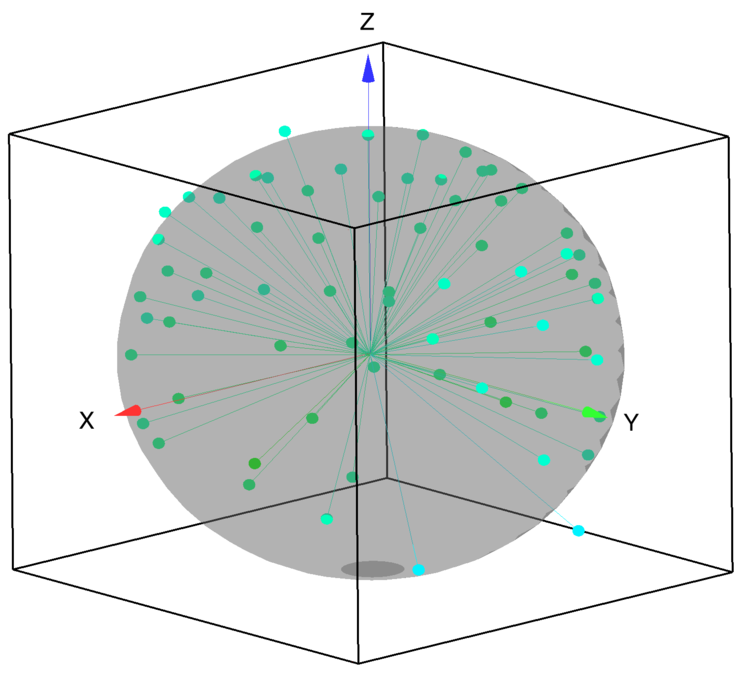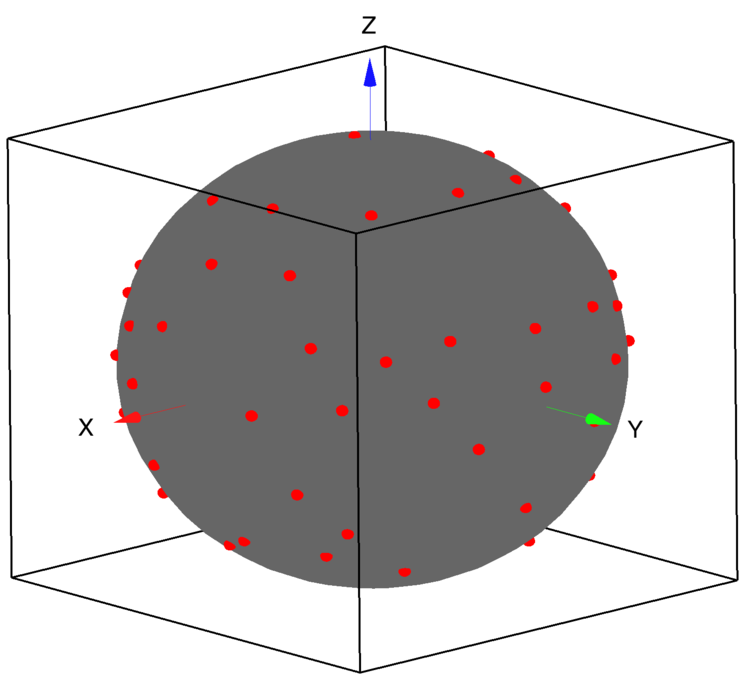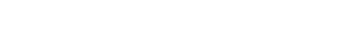# How to compute and display scalp potentials from EEG recordings

EEG researchers commonly create spatial maps of the electrical activity acros the scalp. Data are interpolated or triangulated from a discrete number of electrode placements distributed across the scalp (generally greater than 32 sites) often in fixed/standardized positions (see, e.g., http://www.ant-neuro.com/products/waveguard_original).

To do this, electrode coordinates, either as {x, y, z} triplets or in spherical coordinates are needed and can be obtained from the manufacturer of electrode caps (e.g., https://www.egi.com/knowledge-center/item/71-sensor-position-files-for-visualizing-data-in-third-party-software).

Theoretical analysis typically involves modeling the head as a perfect sphere.  The mapping of the electrode coordinates to the surface of a sphere can be accomplished with the following function:

Function scaleToSpherical(inWave)
Wave inWave

// offset the coordinates to the  origin:
MatrixOP/o/free wave0c=subtractMean(inWave,1)

// compute the distance of electrodes from the origin:

// rescale the distance to a radius of 1:
EndFigure 1:Initial electrode positions relative to the surface of a sphere.Figure 2: Normalized electrode coordinates on the surface of a sphere.

Using the normalized set of coordinates we can triangulate the data on the surface of a sphere using the SphericalTriangulate operation.

`SphericalTriangulate centeredNormalized // this creates M_TriangulationData`

Following the triangulation we can use the wave M_TriangulationData combined with the EEG data to interpolate the potentials at any point on the sphere.  In order to display the resulting interpolation in Gizmo we start by creating a parametric surface representing the sphere.

Function makeParametricSphere(pointsx,pointsy)
Variable pointsx,pointsy

Make/O/N=(pointsx,pointsy,3) parametricW
Variable anglePhi,angleTheta
Variable dPhi,dTheta

dPhi=2*pi/(pointsx-1)
dTheta=pi/(pointsy-1)
Variable xx,yy,zz
Variable sig

for(j=0;j<pointsy;j+=1)
angleTheta=j*dTheta
zz=sin(angleTheta)
if(angleTheta>pi/2)
sig=-1
else
sig=1
endif
for(i=0;i<pointsx;i+=1)
anglePhi=i*dPhi
xx=zz*cos(anglePhi)
yy=zz*sin(anglePhi)
parametricW[i][j]=xx
parametricW[i][j]=yy
parametricW[i][j]=sig*sqrt(1-xx*xx-yy*yy)
endfor
endfor
End

To complete the interpolation we need to construct one more wave: dataPointsWave.  This wave has 4-columns consisting of the electrode coordinates in the first three columns and the potentials measured by each electrode at some fixed time t0 in the fourth column:

Variable nRows=DimSize(centeredNormalized,0)
Make/O/D/N=(nRows,4) dataPointsWave
dataPointsWave[][0,2]=centeredNormalized
dataPointsWave[]=electrodePotentials[p]

The interpolation is computed by:

`SphericalInterpolate M_TriangulationData,dataPointsWave,sphereData`

The resulting interpolation is stored in the wave W_SphericalInterpolation where each row contains the scalar potential interpolated for the corresponding XYZ location in the wave sphereData.  To display these values on the parametric surface we need to construct a color wave

Function createParametricColorWave(pWave,interpWave,reverseCTAB)
Wave pWave,interpWave
Variable reverseCTAB            // set to 1 to reverse; 0 otherwise

// the color wave must match the parametric surface:
Variable rows=dimsize(pwave,0)
Variable cols=dimSize(pWave,1)

// Create the parametric color wave:
Make/O/N=(rows,cols,4) pWaveColor=1

// find the range of values for scaling:
Variable mmax=WaveMax(interpWave)
Variable mmin=WaveMin(interpWave)

// optionally replace the colortable here:
ColorTab2Wave rainbow256
Wave M_Colors
// not all colortables have the same number of colors:
Variable nTableCols=DimSize(M_Colors,0)-1

if(reverseCTAB)
MatrixOP/o/free aa=col(M_Colors,0)
WaveTransform/o flip aa
MatrixOP/O M_Colors=setCol(M_Colors,0,aa)
MatrixOP/o/free aa=col(M_Colors,1)
WaveTransform/o flip aa
MatrixOP/O M_Colors=setCol(M_Colors,1,aa)
MatrixOP/o/free aa=col(M_Colors,2)
WaveTransform/o flip aa
MatrixOP/O M_Colors=setCol(M_Colors,2,aa)
endif

MatrixOP/O M_Colors=fp32(M_Colors/65535)
Variable nor=nTableCols/(mmax-mmin)
Variable i, np=rows*cols,index,rr,cc
for(i=0;i<np;i+=1)
index=trunc((interpWave[i]-mmin)*nor)
rr=mod(i,rows)
cc=trunc(i/rows)
pWaveColor[rr][cc]=M_Colors[index]
pWaveColor[rr][cc]=M_Colors[index]
pWaveColor[rr][cc]=M_Colors[index]
endfor
End

An example of this approach is illustrated in a cross-lingustic speech discrimination study at the following webpage:

Valerie L. Shafer, Ph.D.
vshafer@gc.cuny.edu
Professor and Deputy Executive Officer
Ph.D. Program in Speech-Language-Hearing Sciences
365 Fifth Avenue, NY, NY 10016ForumSupportGallery